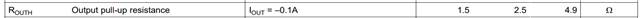If you have a related question, please click the "Ask a related question" button in the top right corner. The newly created question will be automatically linked to this question.

# UCC21750QDWEVM-025: About Measuring Output Pull-up resistance

Part Number: UCC21750QDWEVM-025
Other Parts Discussed in Thread: UCC21750-Q1, UCC21750

Hello, I'm studying about Gate Driver IC operation through several measuring test.

In the datasheet of UCC21750-Q1, Output Pull-up resistance value is written as 2.5ohm when output current is -0.1A (9page of UCC21750-Q1 datasheet)

1) Output current = -0.1A, negative means sourcing from Gate Driver IC to Power Tr. ? Loading current in GATE PAD.

2) I connected the current load to OUTH(TP12) after connecting VCC=5V, VDD=15V, COM=VE=0V, IN+=5V, IN-=0V and measured OUTH volatage it when loading currentis -0.1A, the OUTH was 14.5V.

Calculating the resistance from VDD to OUTH, Output Pull-up resistance, we can see (15V-14.5V)/0.1A = 5ohms, which is different from 2.5ohms in datasheet.

I thought the on resistance of PMOS in the hybrid pull-up system was measured, and I don't understand that the value was different.

as my calculation based on the information in datasheet, PMOS=2.5ohm/ Total resistance (PMOS parallel NMOS) = 0.6ohm/ NMOS=0.78ohm. Is it right?

Please let me know if there's anything I didn't think of. Thank you.

Best regard,

Yoonjin Kim

• Hi Yoonjin,

1. Yes, negative current means the current is sourced from the IC pin; current flowing out of a pin will have a negative sign.

2. The datasheet specified that the typical value is 2.5ohms and the maximum value is 4.9ohms. Depending on the test condition and accuracy of the measurement tools, I suspect your measurement is closer to the upper end of the datasheet spec, thus closer to 4.9ohms.

In the hybrid pull-up structure, the resistance measured is usually the parallel resistance of the NMOS and PMOS. At a high Vouth (the test condition with Iout=-0.1A), the NMOS is very weakly turned on, if it's turned on at all. Thus, what you measured at this test condition is close to the turn on resistance of PMOS. We will actually have an FAQ regarding the hybrid pull-up structure soon, so stay tuned!

Thanks,

Vivian

I have a question.

1)Where can I find the maximum value 4.9ohm, pull-up resistance value specified on datasheet?

As I know there is only typical value specified.

• And also, below the page 9 of UCC21750-Q1 datasheet, it says 2.5ohm is 'Internal PMOS only'.

If maximum value of this item is 4.9ohm, then ON resistance of PMOS could be varying from 2.5ohm to 5ohm?

That big difference will have a big impact on the switching speed, so I wonder if I understand it.

Thank you for your kind response.

Sincerely,

Yoonjin

• Hi YoonJin,

See below on the UCC21750 datasheet that talks about the min, typical and max output pull-up resistance values:This output pull-up resistance is measured at Iout = -0.1A, which is when the gate is almost charged to VDD. The switching speed mostly depends on the peak current, which is specified at 10A. When the output is at peak current, the pull-up resistance will equal to the parallel resistance of the NMOS and PMOS, which is a much smaller value.

Thanks,

Vivian

• Thank you. Vivian.

By the way, I thought the gate driver ic which is mounted on the EVB is UCC21750-Q1.

So I was looking at the datasheet of UCC21750-Q, and only the typ. value whas shown there.

Can I ask why the values of UCC21750 and UCC21750-Q are different? I thought the chip configuration was the same, but I'm curious.

Can I proceed with the test referring to the data sheet of UCC21750?

• Hi YoonJin,

Interesting - I did not notice that these two parts have different datasheet values. My guess would be it has something to do with the AEC-Q100 automotive qualification criteria; I can ask my colleague about that. In this case, I would recommend using UCC21750's output pull-up resistance as a reference but do not count on that, since it's not on the UCC21750-Q1's datasheet.

If this answers your question let me know by pressing the green button, and feel free to reach out again if you have other questions!

Thanks,

Vivian